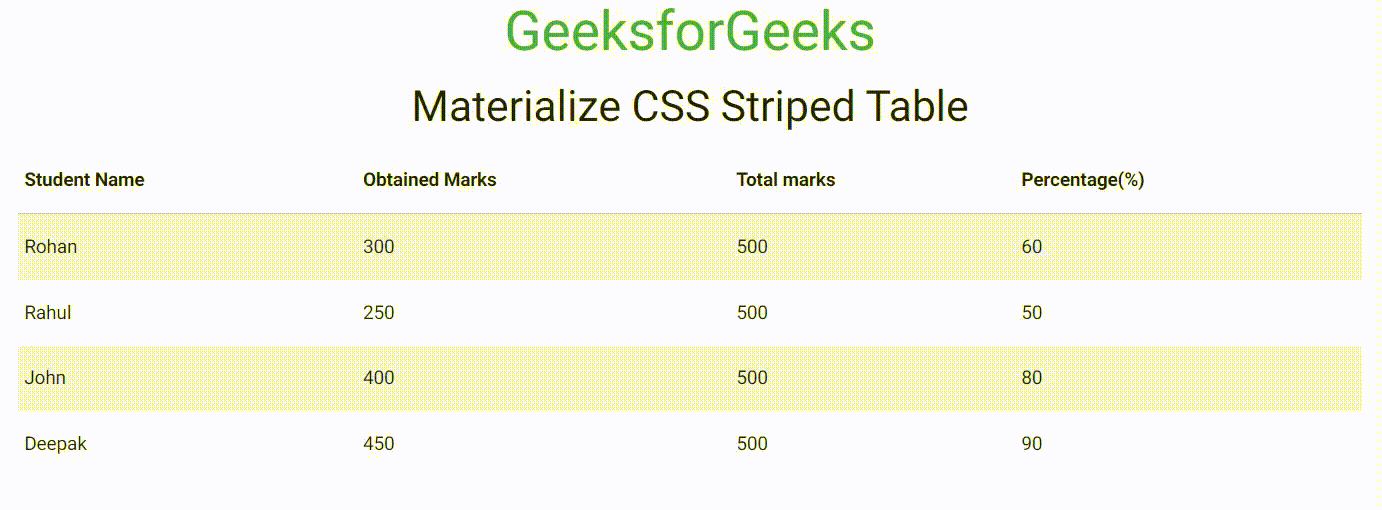GFG App
Open AppBrowser
Continue

# Materialize CSS Striped Table

Materialize CSS is a design language that combines the classic principles of successful design along with innovation and technology. It is created and designed by Google. It is very easy to use to make our webpage dynamic and responsive.

Materialize CSS Table is used to store the data in the rows and columns in a well-structured manner. Materialize CSS Striped Table is used to create the striped table with the help of striped class. In this article, we will discuss Striped Table in Materialize CSS.

Materialize CSS Striped Table Class:

• striped: This class is used to give the strip style to the table.

Syntax:

```<table class="striped">
...
</table>```

Example: The following code demonstrates the Materialize CSS Striped Table.

## HTML

 ` ` `<``html``> ` ` `  `<``head``> ` `    ``` `    ``<``link` `rel``=``"stylesheet"` `href``= ` `"https://cdnjs.cloudflare.com/ajax/libs/materialize/0.97.5/css/materialize.min.css"``> ` ` `  `    ``` `    ``<``meta` `name``=``"viewport"` `content``= ` `        ``"width=device-width, initial-scale=1.0"` `/> ` ` ` ` `  `<``body``> ` `    ``<``div` `class``=``"container center-align"``> ` `        ``<``h3` `class``=``"green-text"``> GeeksforGeeks ` `        ``<``h4``> Materialize CSS Striped Table ` ` `  `        ``<``table` `class``=``"striped"``> ` `            ``<``thead``> ` `                ``<``tr``> ` `                    ``<``th``> Student Name ` `                    ``<``th``> Obtained Marks ` `                    ``<``th``> Total marks ` `                    ``<``th``> Percentage(%) ` `                `` ` `            `` ` ` `  `            ``<``tbody``> ` `                ``<``tr``> ` `                    ``<``td``>Rohan ` `                    ``<``td``> 300 ` `                    ``<``td``> 500 ` `                    ``<``td``> 60 ` `                `` ` `                ``<``tr``> ` `                    ``<``td``> Rahul ` `                    ``<``td``> 250 ` `                    ``<``td``> 500 ` `                    ``<``td``> 50 ` `                `` ` `                ``<``tr``> ` `                    ``<``td``> John ` `                    ``<``td``> 400 ` `                    ``<``td``> 500 ` `                    ``<``td``> 80 ` `                `` ` `                ``<``tr``> ` `                    ``<``td``> Deepak ` `                    ``<``td``> 450 ` `                    ``<``td``> 500 ` `                    ``<``td``> 90 ` `                `` ` `            `` ` `        `` ` `    `` ` ` ` ` `  ``

Output:Materialize CSS Striped Table

My Personal Notes arrow_drop_up